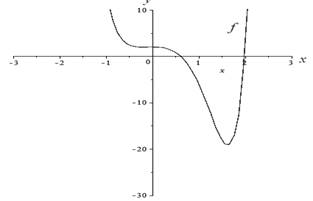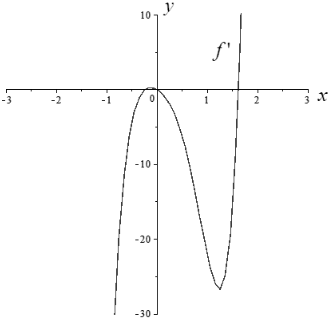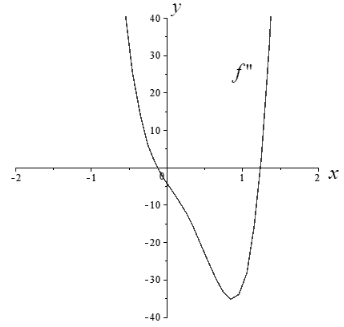# The intervals where the extreme value is increasing and decreasing.### Single Variable Calculus: Concepts...

4th Edition
James Stewart
Publisher: Cengage Learning
ISBN: 9781337687805### Single Variable Calculus: Concepts...

4th Edition
James Stewart
Publisher: Cengage Learning
ISBN: 9781337687805

#### Solutions

Chapter 4, Problem 17RE
To determine

## To find: The intervals where the extreme value is increasing and decreasing.

Expert Solution

### Explanation of Solution

Given information:

The function is f(x)=3x65x5+x45x32x2+2 .

Calculation:

Consider the equation.

f(x)=f(x)=3x65x5+x45x32x2+2

Differentiate above equation.

f(x)=18x525x4+4x315x24x

And,

f(x)=90x4100x3+12x230x4

The graph f(x) is shown figure-1Figure-1

The absolute maximum value is f(1)=10

The absolute minimum value is f(1.8)=18

The graph f(x) is shown figure-2Figure-2

The extreme value for f(0.23)=1.96,f(0)=0 and f(1.62)=19.2

The graph f(x) is shown figure-3Figure-3

Therefore the inflection points for f(x) are (0.12,1.98),(1.24,12.1)

### Have a homework question?

Subscribe to bartleby learn! Ask subject matter experts 30 homework questions each month. Plus, you’ll have access to millions of step-by-step textbook answers!